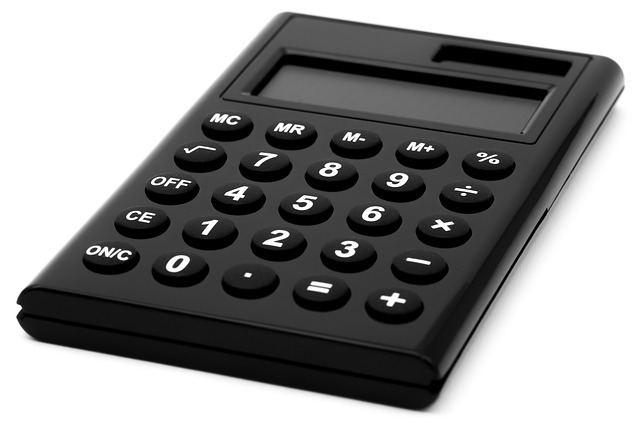AP, IB, and College Microeconomic and Macroeconomic Principles

# 22 Macroeconomics formulas you need to know for ​the Exam

Updated 3/20/2017 Jacob Reed
Below you will find all the formulas you need to know for Macro Economics. Unfortunately for some, the Macro exam is a little more math heavy than the Micro exam. But fear not, the list below is all you need.GDP Formulas
Output Expenditure Model
GDP=C+Ig+G+(X-M)= Consumption + Gross Investment + Government Spending+ (Exports – Imports)
Income Approach
GDP = Compensation of Employees + Rents + Interest + Proprietors Income + Corporate Profits + Taxes on Imports + Statistical Discrepancy – Consumption of Fixed Capital

Time Value of Money
Future Value = Present Value + (Present Value x Interest Rate)
Present Value ≈ Future Value – (Future Value x Interest Rate)